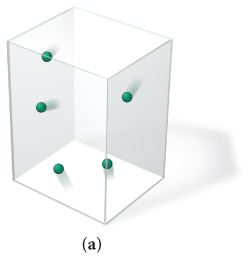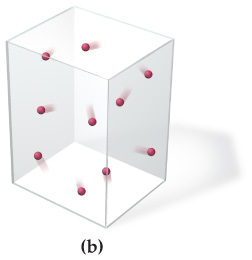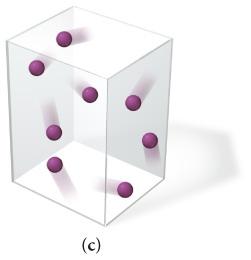# Problem: Which gas sample has the greatest pressure? Assume that all the samples are at the same temperature.

###### FREE Expert Solution

We have to calculate which of the given gas samples has the highest pressure.

We will solve this problem using the ideal gas equation.

Where,

P = pressure

V = volume

n = number of moles

R = gas constant = 0.08206 L•atm/mol•K

T = temperature in Kelvin units

82% (144 ratings)###### Problem Details

Which gas sample has the greatest pressure? Assume that all the samples are at the same temperature.